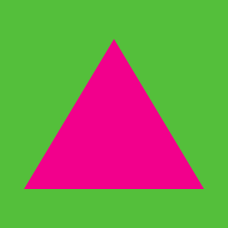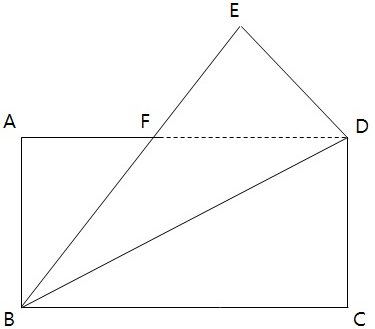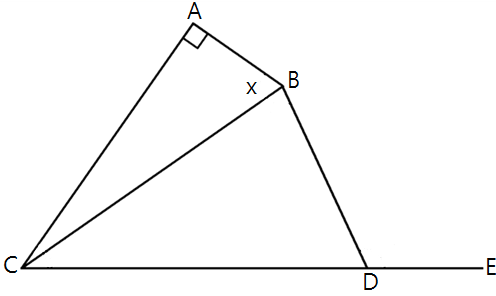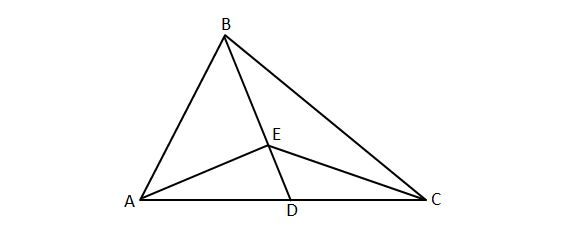Geometry

# Triangles Problem SolvingAs shown in the above diagram, Raj folds a rectangular paper $ABCD$ in such a way that side $BC$ becomes $BE.$ If the length of $\overline{AB}$ is $3$ and the length of $\overline{BC}$ is $15,$ what is the area of $\triangle ABF?$

Note: The above diagram is not drawn to scale.In the above diagram, we are given $\angle BAC=90^\circ, \angle ACD=75^\circ, \angle CBD=75^\circ, \angle BDE=110^\circ.$ What is $\angle ABC$ in degrees?

In right triangle $ABC$, we are given that $\angle ABC = 90^\circ$ and $AC= 34$. $D$ is a point on line segment $BC$ such that $BD=12, DC=18$. What is the length of $AD$?In the above diagram, the ratio of the length of $\overline{AD}$ to the length of $\overline{DC}$ is $3:2.$ If the area of $\triangle ABE$ is $13,$ what is the area of $\triangle BEC ?$

Note: The above diagram is not drawn to scale.

In triangle $ABC$, we have $AB = 47, BC = 48, CA = 49$. Points $D, E,$ and $F$ are the midpoints of $BC, CA,$ and $AB$ respectively. What is the perimeter of triangle $DEF$?

×